Next: Binomial Probability Distribution Up: Probability Theory Previous: Two-State System

# Combinatorial Analysis

The branch of mathematics that studies the number of different ways of arranging things is called combinatorial analysis. We need to know how many different ways there are of arranging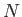objects that are made up of two groups of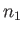and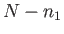indistinguishable objects. This is a rather difficult problem. Let us start off by tackling a slightly easier problem. How many ways are there of arrangingdistinguishable objects? For instance, suppose that we have six pool balls, numbered one through six, and we pot one each into every one of the six pockets of a pool table (that is, top-left, top-right, middle-left, middle-right, bottom-left, and bottom-right). How many different ways are there of doing this? Let us start with the top-left pocket. We could pot any one of the six balls into this pocket, so there are 6 possibilities. For the top-right pocket we only have 5 possibilities, because we have already potted a ball into the top-left pocket, and it cannot be in two pockets simultaneously. So, our 6 original possibilities combined with these 5 new possibilities gives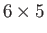ways of potting two balls into the top two pockets. For the middle-left pocket we have 4 possibilities, because we have already potted two balls. These possibilities combined with ourpossibilities gives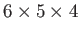ways of potting three balls into three pockets. At this stage, it should be clear that the final answer is going to be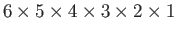. The factorial of a general positive integer,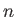, is defined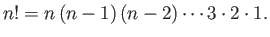(2.17)

So,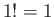, and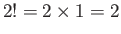, and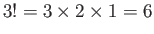, and so on. Clearly, the number of ways of potting six distinguishable pool balls into six pockets is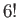(which incidentally equals 720). Because there is nothing special about pool balls, or the number six, we can safely infer that the number of different ways of arrangingdistinguishable objects, denoted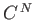, is given by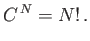(2.18)

Suppose that we take the number four ball off the pool table, and replace it by a second number five ball. How many different ways are there of potting the balls now? Consider a previous arrangement in which the number five ball was potted into the top-left pocket, and the number four ball was potted into the top-right pocket, and then consider a second arrangement that only differs from the first because the number four and five balls have been swapped around. These arrangements are now indistinguishable, and are therefore counted as a single arrangement, whereas previously they were counted as two separate arrangements. Clearly, the previous arrangements can be divided into two groups, containing equal numbers of arrangements, that differ only by the permutation of the number four and five balls. Because these balls are now indistinguishable, we conclude that there are only half as many different arrangements as there were before. If we take the number three ball off the table, and replace it by a third number five ball, then we can split the original arrangements into six equal groups of arrangements that differ only by the permutation of the number three, four, and five balls. There are six groups because there are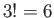separate permutations of these three balls. Because the number three, four, and five balls are now indistinguishable, we conclude that there are only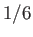the number of original arrangements. Generalizing this result, we conclude that the number of arrangements ofindistinguishable anddistinguishable objects is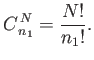(2.19)

We can see that if all the balls on the table are replaced by number five balls then there is only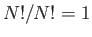possible arrangement. This corresponds, of course, to a number five ball in each pocket. A further straightforward generalization tells us that the number of arrangements of two groups ofandindistinguishable objects is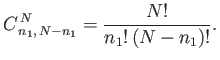(2.20)Next: Binomial Probability Distribution Up: Probability Theory Previous: Two-State System
Richard Fitzpatrick 2016-01-25